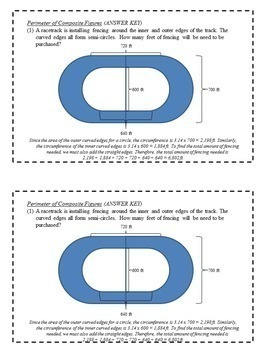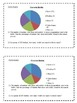Subject
Resource Type
File Type

Zip

(7 MB|188 pages)
Standards
12 Products in this Bundle
12 products
Also included in:
1. This bundle includes over 80 of my individual resources, including math task cards, games, scavenger hunts, review activities, and more for upper elementary and middle school math. Resources can be used during math workshop centers or in a whole group setting. These activities are designed for 6th g
\$158.50
\$98.00
Save \$60.50
2. This bundle includes over 120 of my individual resources, including math task cards, exit slips, board games, scavenger hunts, and math sorts for upper elementary and middle school math. Activities can be use during math workshop centers or as supplementary activities in a whole group setting. Produ
\$325.50
\$185.00
Save \$140.50
• Bundle Description
• Standards

This resource includes 6th grade math enrichment task cards for the 12 different math units shown below. It consists of 1-3 enrichment problems for each of the topics listed below (162 total problems). An answer key IS included! To see a free sample of the type of problems included, download the first unit for free.

Get the majority of my math products, including this full year set of task cards, at a large discount by purchasing them as a part of my Math Workshop Full Year Mega Bundle.

Math Workshop Full Year Mega Bundle (For Upper Elementary/Middle School Math)

Following are the concepts included in this task card bundle.

DECIMALS AND EXPONENTS

(1) Multiply Decimals by Whole Numbers

(2) Multiply Decimals by Decimals

(3) Estimate Quotients

(4) Divide Decimals by Whole Numbers

(5) Divide Decimals by Decimals

(6) Exponents

FRACTION MULTIPLICATION AND DIVISION

(1) Estimate Products of Fractions

(2) Multiply Fractions and Whole Numbers

(3) Multiply Fractions

(4) Multiply Mixed Numbers

(5) Divide Whole Numbers by Fractions

(6) Divide Fractions

(7) Divide Mixed Numbers

RATES AND RATIOS

(1) Ratios

(2) Unit Rates

(3) Ratio Tables

(4) Equivalent Ratios

(5) Ratio and Rate Problems

FRACTIONS, DECIMALS, AND PERCENTS

(1) Decimals as Fractions

(2) Fractions as Decimals

(3) Percents as Fractions

(4) Fractions as Percents

(5) Percents and Decimals

(6) Percents Greater than 100% and Less than 1%

(7) Compare and Order Fractions

(8) Compare and Order Fractions, Decimals, and Percents

(9) Estimate with Percents

(10) Percent of a Number

ALGEBRAIC EXPRESSIONS AND PROPERTIES

(1) Numerical Expressions

(2) Variables and Expressions

(3) Write Expressions

(4) Algebra: Properties

(5) Distributive Property

EQUATIONS

(1) Equations

(2) Solve and Write Addition Equations

(3) Solve and Write Subtraction Equations

(4) Solve and Write Multiplication Equations

(5) Solve and Write Division Equations

(6) Solve and Write Two-Step Equations

FUNCTIONS, INEQUALITIES, AND ABSOLUTE VALUE

(1) Graphing Relations

(2) Function Tables

(3) Function Rules

(4) Functions, Equations, and Graphs

(5) Inequalities

(6) Write and Graph Inequalities

(7) One-Step Inequalities

(8) Two-Step Inequalities

(9) Integers and Absolute Value

(1) Points, Lines, and Planes

(2) Measuring Angles

(3) Angle Relationships

(4) Triangles

(6) Properties of Polygons

(7) Similar and Congruent Figures

(8) Translations

(9) Reflections

(10) Rotations

PERIMETER, AREA, AND VOLUME

(1) Area of Parallelograms

(2) Area of Triangles

(3) Area of Trapezoids

(4) Circumference

(5) Area of Circles

(6) Perimeter of Composite Figures

(7) Area of Composite Figures

(8) Volume of Rectangular Prisms

(9) Surface Area of Rectangular Prisms

VOLUME AND SURFACE AREA

(1) Volume of Triangular Prisms

(2) Volume of Pyramids

(3) Volume of Cylinders

(4) Volume of Cones

(5) Surface Area of Cylinders

(6) Volume of Composite Figures

DATA AND GRAPHS

(1) Mean

(2) Median, Mode, and Range

(3) Appropriate Measures

(4) Frequency Tables

(5) Histograms

(6) Circle Graphs

(7) Appropriate Displays

PROBABILITY

(1) Probability of Simple Events

(2) Sample Spaces

(3) Fundamental Counting Principle

(4) Probability of Independent Events

(5) Probability of Dependent Events

(6) Make Predictions

Each page has two copies of the problems for that topic, making it easy to print, copy, and cut into half pieces of paper for students to use.

Problems are not meant to be a lesson in itself, but can be used in lots of different ways. Great to use in math centers for advanced students, as challenge problems for students who are finished with work, or as exit slips. These challenge problems are intended for use in 6th grade, but could also be used for advanced 5th grade students or 7th grade review.

Purchase units individually by visiting my store! Enjoy!

Be sure to also check out my Math Task Cards at the following link. These are a more basic version of the Math Enrichment Task Cards.

Solve unit rate problems including those involving unit pricing and constant speed. For example, if it took 7 hours to mow 4 lawns, then at that rate, how many lawns could be mowed in 35 hours? At what rate were lawns being mowed?
Make tables of equivalent ratios relating quantities with whole-number measurements, find missing values in the tables, and plot the pairs of values on the coordinate plane. Use tables to compare ratios.
Use ratio and rate reasoning to solve real-world and mathematical problems, e.g., by reasoning about tables of equivalent ratios, tape diagrams, double number line diagrams, or equations.
Understand the concept of a unit rate 𝘢/𝘣 associated with a ratio 𝘢:𝘣 with 𝘣 ≠ 0, and use rate language in the context of a ratio relationship. For example, “This recipe has a ratio of 3 cups of flour to 4 cups of sugar, so there is 3/4 cup of flour for each cup of sugar.” “We paid \$75 for 15 hamburgers, which is a rate of \$5 per hamburger.”
Understand the concept of a ratio and use ratio language to describe a ratio relationship between two quantities. For example, “The ratio of wings to beaks in the bird house at the zoo was 2:1, because for every 2 wings there was 1 beak.” “For every vote candidate A received, candidate C received nearly three votes.”
Total Pages
188 pages
Included
Teaching Duration
N/A
Report this Resource to TpT
Reported resources will be reviewed by our team. Report this resource to let us know if this resource violates TpT’s content guidelines.